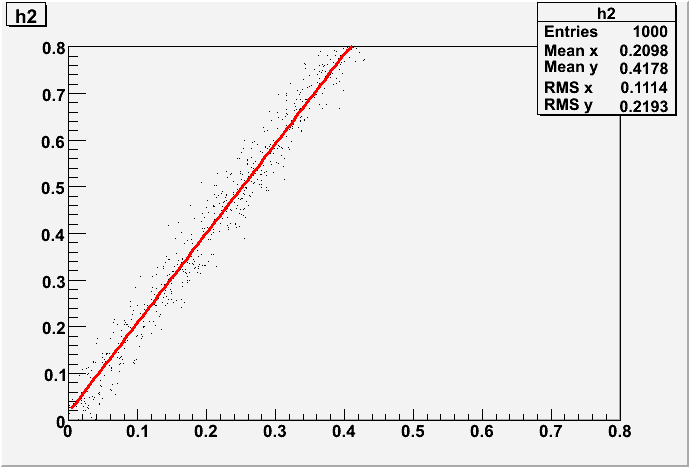# Fitting 2D Histograms

Hello,
I have some problems in fitting a 2D histogram. I attached an example of the kind of histogram I’m dealing with.
Can you help me?
Valentina
angolivsalignedarray.pdf (59.6 KB)

which kind of fit you would like to to ? If you want to see a trend like an angle vs some other variable, it is maybe better to make a profile (TProfile class) and fit it with a 1D function.

Cheers, Lorenzo

Lorenzo,

Note that I recently implemented (SVN trunk) the possibility to fit directly a TH2 with a TF1 without producing an intermediate TProfile.

Rene

Rene,
Regards.
Aous

see example below

``````void f12() {
Double_t angle = TMath::Pi()/4.;
Double_t cosphi = TMath::Cos(angle);
Double_t sinphi = TMath::Sin(angle);

TH2F *h2 = new TH2F("h2","h2",100,0,0.8,100,0,0.8);
TRandom r;
for (Int_t i=0;i<1000;i++) {
Double_t x = r.Uniform(0,1);
Double_t y = r.Gaus(0,0.02);
Double_t u = cosphi*x -sinphi*y;
Double_t v = sinphi*x +cosphi*y;
h2->Fill(u,v);
}
TF1 *f1 = new TF1("f1","+*x",0,1);
f1->SetParameters(0.,1.);
f1->SetLineColor(kRed);
h2->Fit(f1);
h2->Draw();
f1->Draw("same");
}
``````

Rene

Rene.
I ran the code you attached and I keep getting this message:
Error in TH2F::Fit: function f1 dimension, 1, does not match histogram dimension, 2

Another thing, when I chnage the histogram, say by multiplying the y value by 2 (v in the code) teh fit function does not seem to match the histogram. I attach a plot showing what I got and the modified code.
c1.ps (19.5 KB)
temp.C (550 Bytes)

It is likely that you are using an old version of ROOT. With 5.18 or newer, I get the following picture when executing your script

Rene